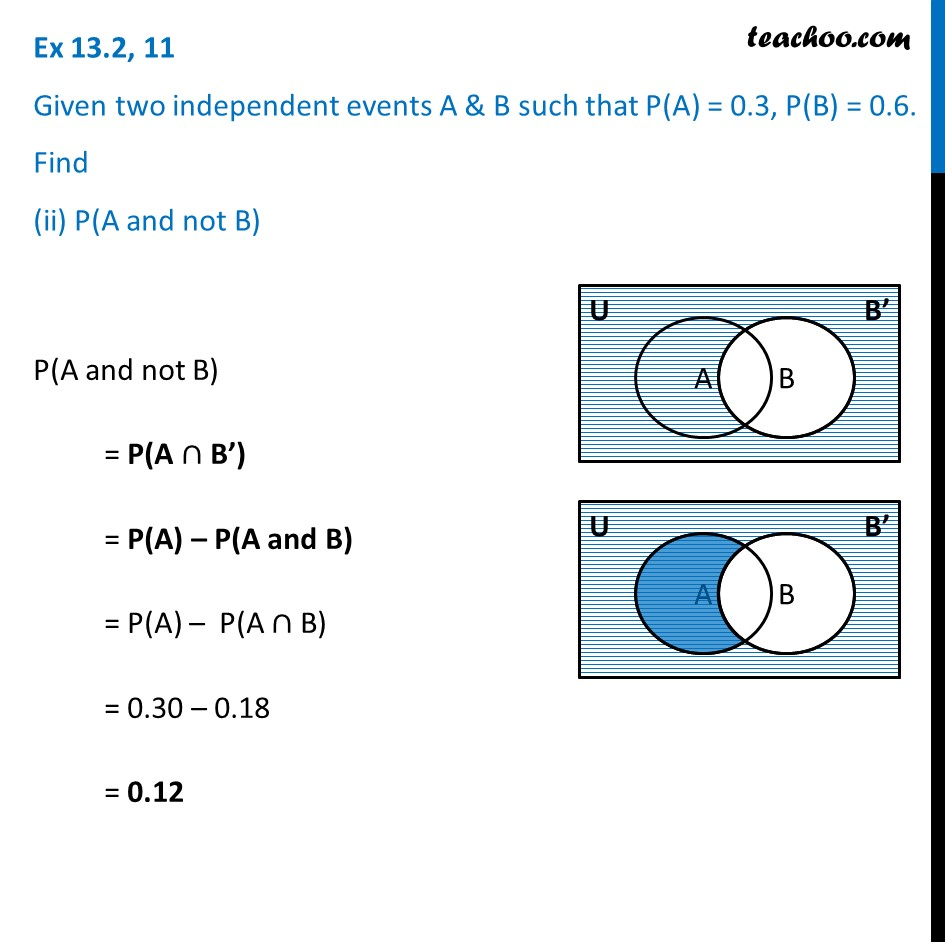Ex 13.2

Chapter 13 Class 12 Probability
Serial order wiseLearn in your speed, with individual attention - Teachoo Maths 1-on-1 Class

### Transcript

Ex 13.2, 11 Given two independent events A & B such that P(A) = 0.3, P(B) = 0.6. Find (ii) P(A and not B) P(A and not B) = P(A ∩ B’) = P(A) – P(A and B) = P(A) – P(A ∩ B) = 0.30 – 0.18 = 0.12